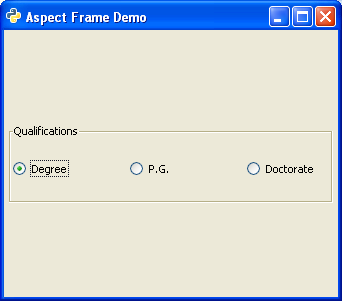# PyGTK - AspectFrame Class

gtk.AspectFrame class is a subclass of the Frame class. The child widget in this frame always retains its aspect ratio (of width and height) even if the main window is resized.

The ratio property of gtk.AspectFrame widget determines the widget width:height ratio. An aspect ratio of 0.5 means the width is one half the height; an aspect ratio of 2.0 means the width is twice the height. The default value for the "ratio" property is 1.0.

The following syntax is used for the constructor of gtk.AspectFrame class −

```gtk.AspectFrame (label = None, xalign = 0.5, yalign = 0.5, ratio = 1.0, obey_child = True)
```

The xalign property determines the fraction of horizontal free space to the left of the child. 0.0 means no free space to the left, 1.0 means all free space to the left.

The yalign property determines the fraction of vertical free space above the child. 0.0 means no free space above, 1.0 means all free space above.

Ratio of width to height of frame is maintained if obey_child property is False.

The obey_child property determines if the ratio is to be ignored. The default is True.

The following code is similar to the one used for the Frame class. The only difference is that the ButonBox is placed in an AspectFrame widget.

```frm = gtk.AspectFrame(label = None, xalign = 0.5, yalign = 0.5,
ratio = 5.0, obey_child = False)
```

Note − The obey_child property is set to False because it is desired to retain the aspect ratio even if the window is resized.

## Example

Observe the following code −

```import gtk

class PyApp(gtk.Window):
def __init__(self):
super(PyApp, self).__init__()
self.set_title("Aspect Frame Demo")
self.set_default_size(250, 200)
self.set_border_width(5)
frm = gtk.AspectFrame(label = None, xalign = 0.5, yalign = 0.5,
ratio = 5.0, obey_child = False)
hb = gtk.HButtonBox()
frm.set_label("Qualifications")
self.connect("destroy", gtk.main_quit)
self.show_all()

if __name__ == '__main__':
PyApp()
gtk.main()
```

The above code will produce the following original and resized windows −Original WindowResized Window